# A4 Classification of Hand-Drawn Digits¶

• A4.1: Now includes optimizers.py
• A4.2: Added A4grader.zip and extra credit.

In this assignment, you will define a new class named NeuralNetworkClassifier that extends the NeuralNetwork class provided here and is the solution to Assignment A2. You will use NeuralNetworkClassifier to train a classifier of hand-drawn digits.

You will also define the function confusion_matrix.

## NeuralNetwork class¶

In :
import matplotlib.pyplot as plt


The following code cell will write its contents to optimizers.py so the import optimizers statement in the code cell after it will work correctly.

In :
%%writefile optimizers.py
import numpy as np

######################################################################
## class Optimizers()
######################################################################

class Optimizers():

def __init__(self, all_weights):
'''all_weights is a vector of all of a neural networks weights concatenated into a one-dimensional vector'''

self.all_weights = all_weights

# The following initializations are only used by adam.
# Only initializing m, v, beta1t and beta2t here allows multiple calls to adam to handle training
# with multiple subsets (batches) of training data.
self.mt = np.zeros_like(all_weights)
self.vt = np.zeros_like(all_weights)
self.beta1 = 0.9
self.beta2 = 0.999
self.beta1t = 1
self.beta2t = 1

def sgd(self, error_f, gradient_f, fargs=[], n_epochs=100, learning_rate=0.001, verbose=True, error_convert_f=None):
'''
error_f: function that requires X and T as arguments (given in fargs) and returns mean squared error.
gradient_f: function that requires X and T as arguments (in fargs) and returns gradient of mean squared error
with respect to each weight.
error_convert_f: function that converts the standardized error from error_f to original T units.
'''

error_trace = []
epochs_per_print = n_epochs // 10

for epoch in range(n_epochs):

error = error_f(*fargs)

# Update all weights using -= to modify their values in-place.

if error_convert_f:
error = error_convert_f(error)
error_trace.append(error)

if verbose and ((epoch + 1) % max(1, epochs_per_print) == 0):
print(f'sgd: Epoch {epoch+1:d} Error={error:.5f}')

return error_trace

'''
error_f: function that requires X and T as arguments (given in fargs) and returns mean squared error.
gradient_f: function that requires X and T as arguments (in fargs) and returns gradient of mean squared error
with respect to each weight.
error_convert_f: function that converts the standardized error from error_f to original T units.
'''

alpha = learning_rate  # learning rate called alpha in original paper on adam
epsilon = 1e-8
error_trace = []
epochs_per_print = n_epochs // 10

for epoch in range(n_epochs):

error = error_f(*fargs)

self.mt[:] = self.beta1 * self.mt + (1 - self.beta1) * grad
self.vt[:] = self.beta2 * self.vt + (1 - self.beta2) * grad * grad
self.beta1t *= self.beta1
self.beta2t *= self.beta2

m_hat = self.mt / (1 - self.beta1t)
v_hat = self.vt / (1 - self.beta2t)

# Update all weights using -= to modify their values in-place.
self.all_weights -= alpha * m_hat / (np.sqrt(v_hat) + epsilon)

if error_convert_f:
error = error_convert_f(error)
error_trace.append(error)

if verbose and ((epoch + 1) % max(1, epochs_per_print) == 0):

return error_trace

if __name__ == '__main__':

import matplotlib.pyplot as plt
plt.ion()

def parabola(wmin):
return ((w - wmin) ** 2)

return 2 * (w - wmin)

w = np.array([0.0])
optimizer = Optimizers(w)

wmin = 5
n_epochs=500, learning_rate=0.1)

print(f'sgd: Minimum of parabola is at {wmin}. Value found is {w}')

w = np.array([0.0])
optimizer = Optimizers(w)
n_epochs=500, learning_rate=0.1)

print(f'adam: Minimum of parabola is at {wmin}. Value found is {w}')

Overwriting optimizers.py

In :
import numpy as np
import optimizers
import sys  # for sys.float_info.epsilon

######################################################################
## class NeuralNetwork()
######################################################################

class NeuralNetwork():

def __init__(self, n_inputs, n_hiddens_per_layer, n_outputs, activation_function='tanh'):
self.n_inputs = n_inputs
self.n_outputs = n_outputs
self.activation_function = activation_function

# Set self.n_hiddens_per_layer to [] if argument is 0, [], or 
if n_hiddens_per_layer == 0 or n_hiddens_per_layer == [] or n_hiddens_per_layer == :
self.n_hiddens_per_layer = []
else:
self.n_hiddens_per_layer = n_hiddens_per_layer

# Initialize weights, by first building list of all weight matrix shapes.
n_in = n_inputs
shapes = []
for nh in self.n_hiddens_per_layer:
shapes.append((n_in + 1, nh))
n_in = nh
shapes.append((n_in + 1, n_outputs))

# self.all_weights:  vector of all weights
# self.Ws: list of weight matrices by layer
self.all_weights, self.Ws = self.make_weights_and_views(shapes)

# Define arrays to hold gradient values.
# One array for each W array with same shape.

self.trained = False
self.total_epochs = 0
self.error_trace = []
self.Xmeans = None
self.Xstds = None
self.Tmeans = None
self.Tstds = None

def make_weights_and_views(self, shapes):
# vector of all weights built by horizontally stacking flatenned matrices
# for each layer initialized with uniformly-distributed values.
all_weights = np.hstack([np.random.uniform(size=shape).flat / np.sqrt(shape)
for shape in shapes])
# Build list of views by reshaping corresponding elements from vector of all weights
# into correct shape for each layer.
views = []
start = 0
for shape in shapes:
size =shape * shape
views.append(all_weights[start:start + size].reshape(shape))
start += size
return all_weights, views

# Return string that shows how the constructor was called
def __repr__(self):
return f'{type(self).__name__}({self.n_inputs}, {self.n_hiddens_per_layer}, {self.n_outputs}, \'{self.activation_function}\')'

# Return string that is more informative to the user about the state of this neural network.
def __str__(self):
result = self.__repr__()
if len(self.error_trace) > 0:
return self.__repr__() + f' trained for {len(self.error_trace)} epochs, final training error {self.error_trace[-1]:.4f}'

def train(self, X, T, n_epochs, learning_rate, method='sgd', verbose=True):
'''
train:
X: n_samples x n_inputs matrix of input samples, one per row
T: n_samples x n_outputs matrix of target output values, one sample per row
n_epochs: number of passes to take through all samples updating weights each pass
learning_rate: factor controlling the step size of each update
method: is either 'sgd' or 'adam'
'''

# Setup standardization parameters
if self.Xmeans is None:
self.Xmeans = X.mean(axis=0)
self.Xstds = X.std(axis=0)
self.Xstds[self.Xstds == 0] = 1  # So we don't divide by zero when standardizing
self.Tmeans = T.mean(axis=0)
self.Tstds = T.std(axis=0)

# Standardize X and T
X = (X - self.Xmeans) / self.Xstds
T = (T - self.Tmeans) / self.Tstds

# Instantiate Optimizers object by giving it vector of all weights
optimizer = optimizers.Optimizers(self.all_weights)

# Define function to convert value from error_f into error in original T units,
# but only if the network has a single output. Multiplying by self.Tstds for
# multiple outputs does not correctly unstandardize the error.
if len(self.Tstds) == 1:
error_convert_f = lambda err: (np.sqrt(err) * self.Tstds) # to scalar
else:
error_convert_f = lambda err: np.sqrt(err) # to scalar

if method == 'sgd':

fargs=[X, T], n_epochs=n_epochs,
learning_rate=learning_rate,
verbose=True,
error_convert_f=error_convert_f)

fargs=[X, T], n_epochs=n_epochs,
learning_rate=learning_rate,
verbose=True,
error_convert_f=error_convert_f)

else:
raise Exception("method must be 'sgd' or 'adam'")

self.error_trace = error_trace

# Return neural network object to allow applying other methods after training.
#  Example:    Y = nnet.train(X, T, 100, 0.01).use(X)
return self

def relu(self, s):
s[s < 0] = 0
return s

return (s > 0).astype(int)

def forward_pass(self, X):
'''X assumed already standardized. Output returned as standardized.'''
self.Ys = [X]
for W in self.Ws[:-1]:
if self.activation_function == 'relu':
self.Ys.append(self.relu(self.Ys[-1] @ W[1:, :] + W[0:1, :]))
else:
self.Ys.append(np.tanh(self.Ys[-1] @ W[1:, :] + W[0:1, :]))
last_W = self.Ws[-1]
self.Ys.append(self.Ys[-1] @ last_W[1:, :] + last_W[0:1, :])
return self.Ys

# Function to be minimized by optimizer method, mean squared error
def error_f(self, X, T):
Ys = self.forward_pass(X)
mean_sq_error = np.mean((T - Ys[-1]) ** 2)
return mean_sq_error

# Gradient of function to be minimized for use by optimizer method
'''Assumes forward_pass just called with layer outputs in self.Ys.'''
error = T - self.Ys[-1]
n_samples = X.shape
n_outputs = T.shape
delta = - error / (n_samples * n_outputs)
n_layers = len(self.n_hiddens_per_layer) + 1
# Step backwards through the layers to back-propagate the error (delta)
for layeri in range(n_layers - 1, -1, -1):
# gradient of all but bias weights
self.dE_dWs[layeri][1:, :] = self.Ys[layeri].T @ delta
# gradient of just the bias weights
self.dE_dWs[layeri][0:1, :] = np.sum(delta, 0)
# Back-propagate this layer's delta to previous layer
if self.activation_function == 'relu':
delta = delta @ self.Ws[layeri][1:, :].T * self.grad_relu(self.Ys[layeri])
else:
delta = delta @ self.Ws[layeri][1:, :].T * (1 - self.Ys[layeri] ** 2)

def use(self, X):
'''X assumed to not be standardized'''
# Standardize X
X = (X - self.Xmeans) / self.Xstds
Ys = self.forward_pass(X)
Y = Ys[-1]
# Unstandardize output Y before returning it
return Y * self.Tstds + self.Tmeans

In :
X = np.arange(100).reshape((-1, 1))
T = (X - 20) ** 3 / 300000

hiddens = 
nnet = NeuralNetwork(X.shape, hiddens, T.shape)

plt.subplot(1, 2, 1)
plt.plot(nnet.error_trace)

plt.subplot(1, 2, 2)
plt.plot(T, label='T')
plt.plot(nnet.use(X), label='Y')
plt.legend()

Adam: Epoch 25 Error=0.23386

Out:
<matplotlib.legend.Legend at 0x7f5c5430c550>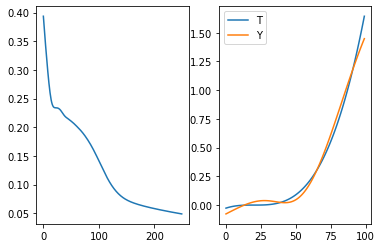## Your NeuralNetworkClassifier class¶

Complete the following definition of NeuralNetworkClassifier as discussed in class. You will need to override the functions

• train
• error_f
• gradient_f
• use

and define the following new functions

• makeIndicatorVars
• softmax
In [ ]:
class NeuralNetworkClassifier(NeuralNetwork):

. . .


Here is a simple test of your new class. For inputs from 0 to 100, classify values less than or equal to 25 as Class Label 25, greater than 25 and less than or equal to 75 as Class Label 75, and greater than 75 as Class Label 100.

In :
X = np.arange(100).reshape((-1, 1))
T = X.copy()
T[T <= 25] = 25
T[np.logical_and(25 < T, T <= 75)] = 75
T[T > 75] = 100

plt.plot(X, T, 'o-')
plt.xlabel('X')
plt.ylabel('Class');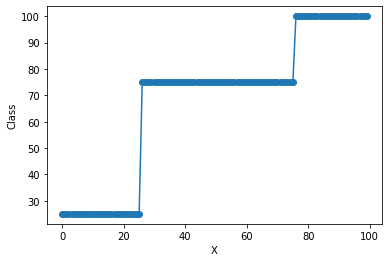In :
hiddens = 
nnet = NeuralNetworkClassifier(X.shape, hiddens, len(np.unique(T)))
nnet.train(X, T, 200, 0.01, method='adam', verbose=True)

plt.subplot(1, 2, 1)
plt.plot(nnet.error_trace)
plt.xlabel('Epoch')
plt.ylabel('Likelihood')

plt.subplot(1, 2, 2)
plt.plot(T + 5, 'o-', label='T + 5')  # to see, when predicted overlap T very closely
plt.plot(nnet.use(X), 'o-', label='Y')
plt.legend()

Adam: Epoch 20 Error=0.78542

Out:
<matplotlib.legend.Legend at 0x7f5c54109250>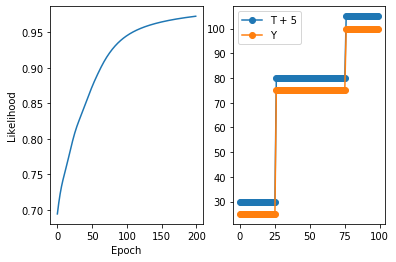## Now for the Hand-Draw Digits¶

We will use a bunch (50,000) images of hand drawn digits from this deeplearning.net site. Download mnist.pkl.gz.

This pickle file includes data already partitioned into training, validation, and test sets. To read it into python, use the following steps

In :
import pickle
import gzip

with gzip.open('mnist.pkl.gz', 'rb') as f:
train_set, valid_set, test_set = pickle.load(f, encoding='latin1')

Xtrain = train_set
Ttrain = train_set.reshape(-1, 1)

Xval = valid_set
Tval = valid_set.reshape(-1, 1)

Xtest = test_set
Ttest = test_set.reshape(-1, 1)

print(Xtrain.shape, Ttrain.shape,  Xval.shape, Tval.shape,  Xtest.shape, Ttest.shape)

(50000, 784) (50000, 1) (10000, 784) (10000, 1) (10000, 784) (10000, 1)

In :
Ttrain[:10]

Out:
array([,
,
,
,
,
,
,
,
,
])

Those must be the digits. What the heck is in those 784 columns in the input matrices?

In :
plt.plot(Xtrain[0, :]);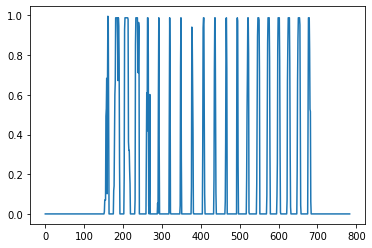Well, values between 0 and 1. That doesn't help much. These are actually intensity values for 784 pixels in an image.

How can we rearrange these values into an image to be displayed? We must first figure out how many columns and rows the image would have. Perhaps the image is a square image, with equal numbers of rows and columns.

In :
import math
math.sqrt(784)

Out:
28.0

Ah, cool.

In :
28 * 28

Out:
784

Ok Let's reshape it and look at the numbers.

In :
image0 = Xtrain[0, :]
image0 = image0.reshape(28, 28)
image0

Out:
array([[0.        , 0.        , 0.        , 0.        , 0.        ,
0.        , 0.        , 0.        , 0.        , 0.        ,
0.        , 0.        , 0.        , 0.        , 0.        ,
0.        , 0.        , 0.        , 0.        , 0.        ,
0.        , 0.        , 0.        , 0.        , 0.        ,
0.        , 0.        , 0.        ],
[0.        , 0.        , 0.        , 0.        , 0.        ,
0.        , 0.        , 0.        , 0.        , 0.        ,
0.        , 0.        , 0.        , 0.        , 0.        ,
0.        , 0.        , 0.        , 0.        , 0.        ,
0.        , 0.        , 0.        , 0.        , 0.        ,
0.        , 0.        , 0.        ],
[0.        , 0.        , 0.        , 0.        , 0.        ,
0.        , 0.        , 0.        , 0.        , 0.        ,
0.        , 0.        , 0.        , 0.        , 0.        ,
0.        , 0.        , 0.        , 0.        , 0.        ,
0.        , 0.        , 0.        , 0.        , 0.        ,
0.        , 0.        , 0.        ],
[0.        , 0.        , 0.        , 0.        , 0.        ,
0.        , 0.        , 0.        , 0.        , 0.        ,
0.        , 0.        , 0.        , 0.        , 0.        ,
0.        , 0.        , 0.        , 0.        , 0.        ,
0.        , 0.        , 0.        , 0.        , 0.        ,
0.        , 0.        , 0.        ],
[0.        , 0.        , 0.        , 0.        , 0.        ,
0.        , 0.        , 0.        , 0.        , 0.        ,
0.        , 0.        , 0.        , 0.        , 0.        ,
0.        , 0.        , 0.        , 0.        , 0.        ,
0.        , 0.        , 0.        , 0.        , 0.        ,
0.        , 0.        , 0.        ],
[0.        , 0.        , 0.        , 0.        , 0.        ,
0.        , 0.        , 0.        , 0.        , 0.        ,
0.        , 0.        , 0.01171875, 0.0703125 , 0.0703125 ,
0.0703125 , 0.4921875 , 0.53125   , 0.68359375, 0.1015625 ,
0.6484375 , 0.99609375, 0.96484375, 0.49609375, 0.        ,
0.        , 0.        , 0.        ],
[0.        , 0.        , 0.        , 0.        , 0.        ,
0.        , 0.        , 0.        , 0.1171875 , 0.140625  ,
0.3671875 , 0.6015625 , 0.6640625 , 0.98828125, 0.98828125,
0.98828125, 0.98828125, 0.98828125, 0.87890625, 0.671875  ,
0.98828125, 0.9453125 , 0.76171875, 0.25      , 0.        ,
0.        , 0.        , 0.        ],
[0.        , 0.        , 0.        , 0.        , 0.        ,
0.        , 0.        , 0.19140625, 0.9296875 , 0.98828125,
0.98828125, 0.98828125, 0.98828125, 0.98828125, 0.98828125,
0.98828125, 0.98828125, 0.98046875, 0.36328125, 0.3203125 ,
0.3203125 , 0.21875   , 0.15234375, 0.        , 0.        ,
0.        , 0.        , 0.        ],
[0.        , 0.        , 0.        , 0.        , 0.        ,
0.        , 0.        , 0.0703125 , 0.85546875, 0.98828125,
0.98828125, 0.98828125, 0.98828125, 0.98828125, 0.7734375 ,
0.7109375 , 0.96484375, 0.94140625, 0.        , 0.        ,
0.        , 0.        , 0.        , 0.        , 0.        ,
0.        , 0.        , 0.        ],
[0.        , 0.        , 0.        , 0.        , 0.        ,
0.        , 0.        , 0.        , 0.3125    , 0.609375  ,
0.41796875, 0.98828125, 0.98828125, 0.80078125, 0.04296875,
0.        , 0.16796875, 0.6015625 , 0.        , 0.        ,
0.        , 0.        , 0.        , 0.        , 0.        ,
0.        , 0.        , 0.        ],
[0.        , 0.        , 0.        , 0.        , 0.        ,
0.        , 0.        , 0.        , 0.        , 0.0546875 ,
0.00390625, 0.6015625 , 0.98828125, 0.3515625 , 0.        ,
0.        , 0.        , 0.        , 0.        , 0.        ,
0.        , 0.        , 0.        , 0.        , 0.        ,
0.        , 0.        , 0.        ],
[0.        , 0.        , 0.        , 0.        , 0.        ,
0.        , 0.        , 0.        , 0.        , 0.        ,
0.        , 0.54296875, 0.98828125, 0.7421875 , 0.0078125 ,
0.        , 0.        , 0.        , 0.        , 0.        ,
0.        , 0.        , 0.        , 0.        , 0.        ,
0.        , 0.        , 0.        ],
[0.        , 0.        , 0.        , 0.        , 0.        ,
0.        , 0.        , 0.        , 0.        , 0.        ,
0.        , 0.04296875, 0.7421875 , 0.98828125, 0.2734375 ,
0.        , 0.        , 0.        , 0.        , 0.        ,
0.        , 0.        , 0.        , 0.        , 0.        ,
0.        , 0.        , 0.        ],
[0.        , 0.        , 0.        , 0.        , 0.        ,
0.        , 0.        , 0.        , 0.        , 0.        ,
0.        , 0.        , 0.13671875, 0.94140625, 0.87890625,
0.625     , 0.421875  , 0.00390625, 0.        , 0.        ,
0.        , 0.        , 0.        , 0.        , 0.        ,
0.        , 0.        , 0.        ],
[0.        , 0.        , 0.        , 0.        , 0.        ,
0.        , 0.        , 0.        , 0.        , 0.        ,
0.        , 0.        , 0.        , 0.31640625, 0.9375    ,
0.98828125, 0.98828125, 0.46484375, 0.09765625, 0.        ,
0.        , 0.        , 0.        , 0.        , 0.        ,
0.        , 0.        , 0.        ],
[0.        , 0.        , 0.        , 0.        , 0.        ,
0.        , 0.        , 0.        , 0.        , 0.        ,
0.        , 0.        , 0.        , 0.        , 0.17578125,
0.7265625 , 0.98828125, 0.98828125, 0.5859375 , 0.10546875,
0.        , 0.        , 0.        , 0.        , 0.        ,
0.        , 0.        , 0.        ],
[0.        , 0.        , 0.        , 0.        , 0.        ,
0.        , 0.        , 0.        , 0.        , 0.        ,
0.        , 0.        , 0.        , 0.        , 0.        ,
0.0625    , 0.36328125, 0.984375  , 0.98828125, 0.73046875,
0.        , 0.        , 0.        , 0.        , 0.        ,
0.        , 0.        , 0.        ],
[0.        , 0.        , 0.        , 0.        , 0.        ,
0.        , 0.        , 0.        , 0.        , 0.        ,
0.        , 0.        , 0.        , 0.        , 0.        ,
0.        , 0.        , 0.97265625, 0.98828125, 0.97265625,
0.25      , 0.        , 0.        , 0.        , 0.        ,
0.        , 0.        , 0.        ],
[0.        , 0.        , 0.        , 0.        , 0.        ,
0.        , 0.        , 0.        , 0.        , 0.        ,
0.        , 0.        , 0.        , 0.        , 0.1796875 ,
0.5078125 , 0.71484375, 0.98828125, 0.98828125, 0.80859375,
0.0078125 , 0.        , 0.        , 0.        , 0.        ,
0.        , 0.        , 0.        ],
[0.        , 0.        , 0.        , 0.        , 0.        ,
0.        , 0.        , 0.        , 0.        , 0.        ,
0.        , 0.        , 0.15234375, 0.578125  , 0.89453125,
0.98828125, 0.98828125, 0.98828125, 0.9765625 , 0.7109375 ,
0.        , 0.        , 0.        , 0.        , 0.        ,
0.        , 0.        , 0.        ],
[0.        , 0.        , 0.        , 0.        , 0.        ,
0.        , 0.        , 0.        , 0.        , 0.        ,
0.09375   , 0.4453125 , 0.86328125, 0.98828125, 0.98828125,
0.98828125, 0.98828125, 0.78515625, 0.3046875 , 0.        ,
0.        , 0.        , 0.        , 0.        , 0.        ,
0.        , 0.        , 0.        ],
[0.        , 0.        , 0.        , 0.        , 0.        ,
0.        , 0.        , 0.        , 0.08984375, 0.2578125 ,
0.83203125, 0.98828125, 0.98828125, 0.98828125, 0.98828125,
0.7734375 , 0.31640625, 0.0078125 , 0.        , 0.        ,
0.        , 0.        , 0.        , 0.        , 0.        ,
0.        , 0.        , 0.        ],
[0.        , 0.        , 0.        , 0.        , 0.        ,
0.        , 0.0703125 , 0.66796875, 0.85546875, 0.98828125,
0.98828125, 0.98828125, 0.98828125, 0.76171875, 0.3125    ,
0.03515625, 0.        , 0.        , 0.        , 0.        ,
0.        , 0.        , 0.        , 0.        , 0.        ,
0.        , 0.        , 0.        ],
[0.        , 0.        , 0.        , 0.        , 0.21484375,
0.671875  , 0.8828125 , 0.98828125, 0.98828125, 0.98828125,
0.98828125, 0.953125  , 0.51953125, 0.04296875, 0.        ,
0.        , 0.        , 0.        , 0.        , 0.        ,
0.        , 0.        , 0.        , 0.        , 0.        ,
0.        , 0.        , 0.        ],
[0.        , 0.        , 0.        , 0.        , 0.53125   ,
0.98828125, 0.98828125, 0.98828125, 0.828125  , 0.52734375,
0.515625  , 0.0625    , 0.        , 0.        , 0.        ,
0.        , 0.        , 0.        , 0.        , 0.        ,
0.        , 0.        , 0.        , 0.        , 0.        ,
0.        , 0.        , 0.        ],
[0.        , 0.        , 0.        , 0.        , 0.        ,
0.        , 0.        , 0.        , 0.        , 0.        ,
0.        , 0.        , 0.        , 0.        , 0.        ,
0.        , 0.        , 0.        , 0.        , 0.        ,
0.        , 0.        , 0.        , 0.        , 0.        ,
0.        , 0.        , 0.        ],
[0.        , 0.        , 0.        , 0.        , 0.        ,
0.        , 0.        , 0.        , 0.        , 0.        ,
0.        , 0.        , 0.        , 0.        , 0.        ,
0.        , 0.        , 0.        , 0.        , 0.        ,
0.        , 0.        , 0.        , 0.        , 0.        ,
0.        , 0.        , 0.        ],
[0.        , 0.        , 0.        , 0.        , 0.        ,
0.        , 0.        , 0.        , 0.        , 0.        ,
0.        , 0.        , 0.        , 0.        , 0.        ,
0.        , 0.        , 0.        , 0.        , 0.        ,
0.        , 0.        , 0.        , 0.        , 0.        ,
0.        , 0.        , 0.        ]], dtype=float32)

Not that helpful. Ok, let's use matplotlib to make an image display.

In :
plt.imshow(image0);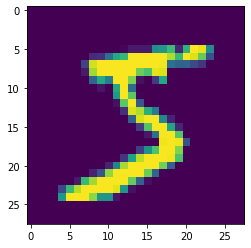Humm. Try a grayscale color map.

In :
plt.imshow(image0, cmap='gray');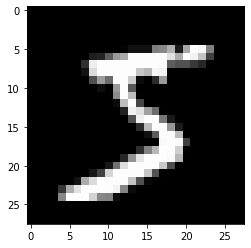With a little more work, we can make it look like a pencil drawing.

In :
plt.imshow(-image0, cmap='gray')  # notice the negative sign
plt.axis('off');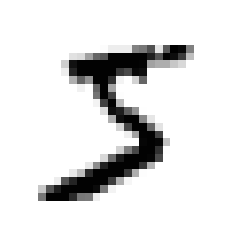Looks like a 5. What class label is associated with this image?

In :
Ttrain

Out:
array()

Okay. Makes sense. Let's look at the first 100 images and their labels, as plot titles.

In :
plt.figure(figsize=(20, 20))
for i in range(100):
plt.subplot(10, 10, i + 1)
plt.imshow(-Xtrain[i, :].reshape(28, 28), cmap='gray')
plt.title(Ttrain[i, 0])
plt.axis('off');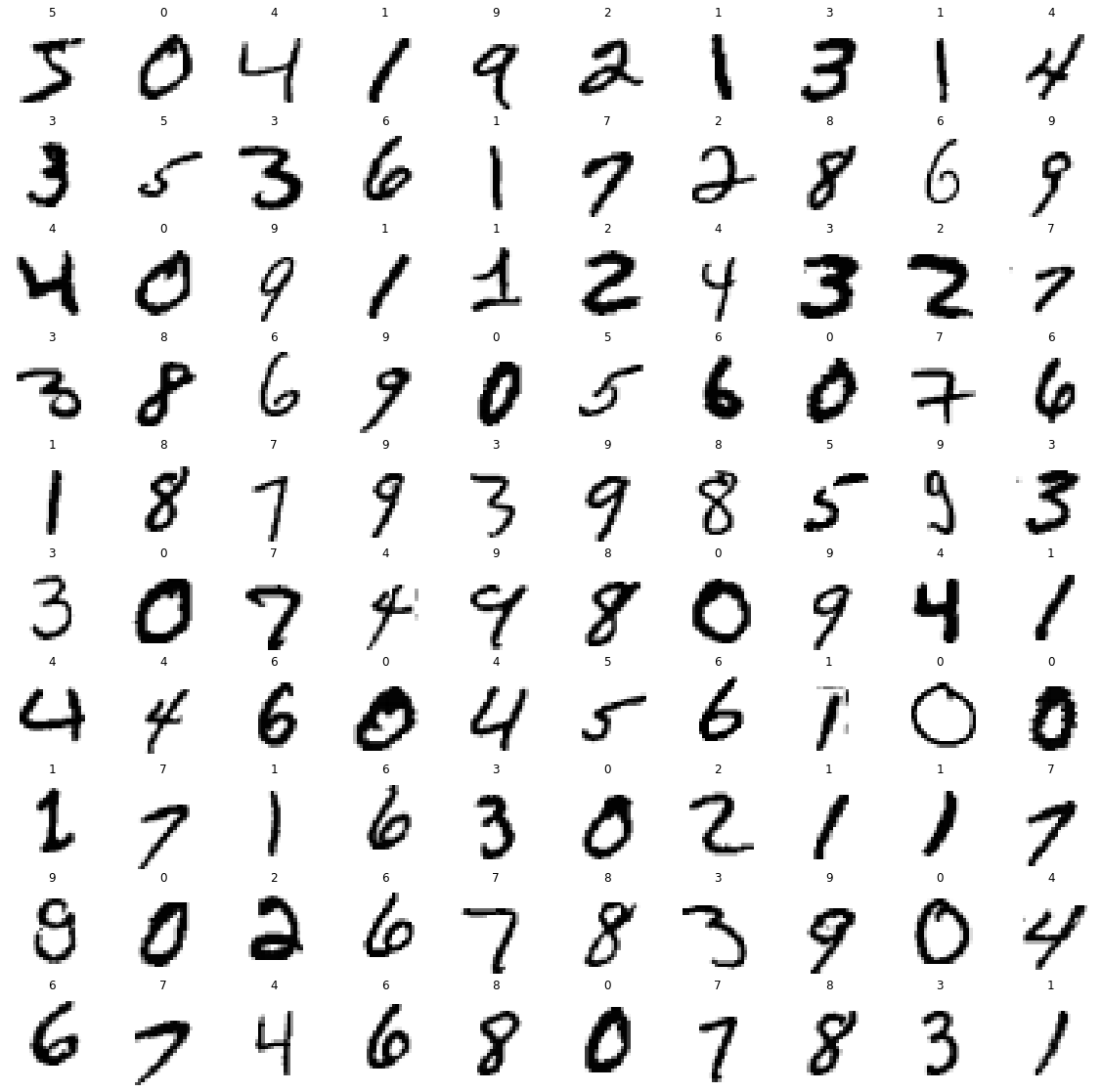Okay. We are ready to try to classify, right?

First we should check the proportions of each digit in the given data partitions.

In :
classes = np.arange(10)
(Ttrain == classes).shape

Out:
(50000, 10)
In :
(Ttrain == classes).sum(axis=0)

Out:
array([4932, 5678, 4968, 5101, 4859, 4506, 4951, 5175, 4842, 4988])
In :
(Ttrain == classes).sum(axis=0) / Ttrain.shape

Out:
array([0.09864, 0.11356, 0.09936, 0.10202, 0.09718, 0.09012, 0.09902,
0.1035 , 0.09684, 0.09976])
In :
['Ttrain', *(Ttrain == classes).sum(axis=0) / Ttrain.shape]

Out:
['Ttrain',
0.09864,
0.11356,
0.09936,
0.10202,
0.09718,
0.09012,
0.09902,
0.1035,
0.09684,
0.09976]
In :
import pandas

result = []
result.append(['Train', *(Ttrain == classes).sum(axis=0) / Ttrain.shape])
result.append(['Tval', *(Tval == classes).sum(axis=0) / Tval.shape])
result.append(['Ttest', *(Ttest == classes).sum(axis=0) / Ttest.shape])
pandas.DataFrame(result)

Out:
0 1 2 3 4 5 6 7 8 9 10
0 Train 0.09864 0.11356 0.09936 0.10202 0.09718 0.09012 0.09902 0.1035 0.09684 0.09976
1 Tval 0.09910 0.10640 0.09900 0.10300 0.09830 0.09150 0.09670 0.1090 0.10090 0.09610
2 Ttest 0.09800 0.11350 0.10320 0.10100 0.09820 0.08920 0.09580 0.1028 0.09740 0.10090

All very close to 0.1. Super.

Time for our first experiment. Let's train a small neural net with 5 hidden units in one layer for a small number of epochs using Adam.

In :
n_epochs = 100
learning_rate = 0.01

np.random.seed(142)

nnet = NeuralNetworkClassifier(Xtrain.shape, , len(classes))
nnet.train(Xtrain, Ttrain, n_epochs, learning_rate, method='adam', verbose=True)

Adam: Epoch 10 Error=0.82887

Out:
NeuralNetworkClassifier(784, , 10, 'tanh')
In :
print(nnet)  # uses the __str__ method

NeuralNetworkClassifier(784, , 10, 'tanh') trained for 100 epochs, final training error 0.9345

In :
plt.plot(nnet.error_trace);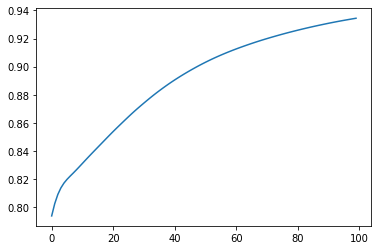Now it is time for you to run some longer experiments. You must write the code to do the following steps:

1. For each of at least five different hidden layer structures

1. Train a network for 500 epochs.
2. Collect percent of samples correctly classified in the given train, validate, and test partitions.
2. Create a pandas.DataFrame with these results and with column headings ('Hidden Layers', 'Train', 'Validate', 'Test', 'Time') where 'Time' is the number of seconds required to train each network.

3. Retrain a network using the best hidden layer structure, judged by the percent correct on the validation set.

4. Use this network to find several images in the test set for which the network's probability of the correct class is the closest to zero, meaning images for which your network does the worst. Draw these images and discuss why your network might not be doing well for those images.

## confusion_matrix¶

Now, write a function named confusion_matrix that returns a confusion matrix for any classification problem, returned as a pandas.DataFrame as shown in Lecture Notes 12. It must require two arguments, the predicted classes for each sample and the true classes for each sample. Here is an example.

In :
Y_classes, Y_probs = nnet.use(Xtest)
confusion_matrix(Y_classes, Ttest)

Out:
0 1 2 3 4 5 6 7 8 9
0 89.897959 0.204082 1.530612 0.000000 1.326531 1.020408 3.877551 1.326531 0.204082 0.612245
1 0.176211 94.625551 1.321586 1.233480 0.264317 0.176211 0.352423 0.088106 1.762115 0.000000
2 1.162791 1.647287 85.658915 2.228682 1.356589 0.387597 3.294574 0.872093 3.100775 0.290698
3 0.000000 0.792079 8.514851 77.821782 0.693069 3.663366 2.376238 1.683168 3.465347 0.990099
4 0.814664 1.120163 1.120163 0.407332 82.179226 1.731161 0.610998 1.018330 0.407332 10.590631
5 2.690583 0.336323 1.233184 3.139013 1.793722 74.887892 4.708520 0.896861 6.726457 3.587444
6 3.131524 0.208768 3.027140 0.626305 0.521921 5.323591 84.237996 2.400835 0.313152 0.208768
7 1.167315 3.015564 1.361868 0.875486 2.918288 0.000000 0.583658 84.241245 0.000000 5.836576
8 1.129363 1.848049 0.821355 6.262834 0.513347 7.905544 1.129363 1.334702 76.591376 2.464066
9 2.081269 1.288404 0.000000 0.991080 5.748266 1.189296 0.000000 3.567889 1.090188 84.043608

In :
%run -i A4grader.py

Testing

nn_reg = NeuralNetwork(1, , 2)
nn_class = NeuralNetworkClassifier(1, , 5)

nn_reg.forward_pass.__func__ == nn_class.forward_pass.__func__

--- 10/10 points.  Function NeuralNetwork.forward_pass is correctly not overridden in NeuralNetworkClassifier.

Testing

nn_reg = NeuralNetwork(1, , 2)
nn_class = NeuralNetworkClassifier(1, , 5)

nn_reg.train.__func__ != nn_class.train.__func__

--- 10/10 points.  Function NeuralNetwork.train is correctly overridden in NeuralNetworkClassifier.

Testing

nn_reg = NeuralNetwork(1, , 2)
nn_class = NeuralNetworkClassifier(1, , 5)

nn_reg.use.__func__ != nn_class.use.__func__

--- 10/10 points.  Function NeuralNetwork.use is correctly overridden in NeuralNetworkClassifier.

Testing

nn_class = NeuralNetworkClassifier(1, , 5)
result = nn_class.makeIndicatorVars(np.arange(5).reshape(-1, 1))

--- 10/10 points.  makeIndicatorVars correctly returned all 1s on diagonal.

Testing

nn_class = NeuralNetworkClassifier(1, , 5)
result = nn_class.softmax(np.array([[-5.5, 5.5]]))

--- 10/10 points.  softmax returned correct answer.

Testing

X = np.arange(20).reshape(20, 1)
X = np.hstack((X, X[::-1, :]))
T = np.array(['ends', 'mid'])[(np.abs(X[:, 0:1] - X[:, 1:2]) < 6).astype(int)]

np.random.seed(42)

nnet = NeuralNetworkClassifier(X.shape, [10, 10], len(np.unique(T)), activation_function='relu')
nnet.train(X, T, 500, 0.001, method='adam', verbose=False)

Y_classes, Y_probs = nnet.use(X)

percent_correct = 100 * np.mean(Y_classes == T)

--- 30/30 points. Correctly returned 100.0.

Testing

cm = confusion_matrix(Y_classes, T)

--- 10/10 points. Correctly returned DataFrame:
ends    mid
ends  100.0    0.0
mid     0.0  100.0

======================================================================
A4 Execution Grade is 90 / 90
======================================================================

__ / 10 Based on other testing and the results you obtain and your discussions.

======================================================================
A4 FINAL GRADE is  _  / 100
======================================================================

Extra Credit:

Earn 1 extra credit point on this assignment by doing the following steps.

1. Combine the train, validate, and test partitions loaded from the MNIST data file into
two matrices, X and T.

2. Using adam , relu and just one value of learning_rate and n_epochs, compare several
hidden layer architectures. Do so by applying our generate_k_fold_cross_validation_sets
function as defined in Lecture Notes 12 which forms stratified partitioning, for use
in classification problems, to your X and T matrices using n_fold of 3.

3. Show results and discuss which architectures you find works the best, and how you
determined this.

A4 EXTRA CREDIT is 0 / 1


## Extra Credit¶

Earn 1 extra credit point on this assignment by doing the following.

1. Combine the train, validate, and test partitions loaded from the MNIST data file into two matrices, X and T.
2. Using adam , relu and just one value of learning_rate and n_epochs, compare several hidden layer architectures. Do so by applying our generate_k_fold_cross_validation_sets function as defined in Lecture Notes 12 which forms stratified partitioning, for use in classification problems, to your X and T matrices using n_fold of 3.
3. Show results and discuss which architectures you find works the best, and how you determined this.